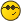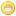A special equation : f^[t](x) = f(t x)/ttommy1729Ultimate FellowPosts: 1,918 Threads: 414 Joined: Feb 2009 06/12/2022, 05:38 PM (This post was last modified: 06/12/2022, 10:34 PM by tommy1729.) Let the t ' th iteration of a real entire function f(x) with f(0) = 0 and f ' (0) = 1 be given by f^[t](x) = 1/n ( f( t^a_1 * x)/(t^a_1) + f( t_a^2 * x)/(t^a_2) + ... + f( t^a_n * x)/(t^a_n) )  for some integer n > 0 and where the a_n are positive reals. for some interval t element of [a,b]. Clearly it automaticly holds for (lim) t = 0 or t = 1. Many related ideas can be made. For instance solve for $f(f(x)) = ( f(2x)/2 + f(4x)/4 )/2.$ *** I had the idea lim f^[-n]( (  f( t f^[n](x) )/t + f( t^2 f^[n](x) )/t^2 )/2 ) as a kind of koenings type function giving f^[t](x) = lim f^[-n]( (  f( t f^[n](x) )/t + f( t^2 f^[n](x) )/t^2 )/2 ) for 0 < t =< 1. regards tommy1729tommy1729Ultimate FellowPosts: 1,918 Threads: 414 Joined: Feb 2009 06/12/2022, 05:44 PM hmm  there is a problem. if f(x) is entire then so is f( 1/2 x). but half iterates are usually not entire. So I guess we are left with special cases and approximations. Still worth investigating though. regards tommy1729tommy1729Ultimate FellowPosts: 1,918 Threads: 414 Joined: Feb 2009 06/12/2022, 10:30 PM (This post was last modified: 06/12/2022, 10:30 PM by tommy1729.) Or perhaps better  with t in interval $[a,b]$ the equation or asymptotic : $f^{[t]}(x)=\sum_{n=1}^K a_n f( t^{2n - 1} x) t^{1-2n},\sum_{n=1}^K a_n = 1$  You know Im starting to get slightly serious when I start using tex. regards tommy1729tommy1729Ultimate FellowPosts: 1,918 Threads: 414 Joined: Feb 2009 06/12/2022, 11:07 PM (06/12/2022, 05:38 PM)tommy1729 Wrote: For instance solve for $f(f(x)) = ( f(2x)/2 + f(4x)/4 )/2.$ ok lets try. f(0) = 0 f(1) = a f(f(1)) = f(a) = f(2)/4 + f(4)/8. f(f(2x)) = f(4x)/4 + f(8x)/8. f(2) = b, f(4) = c, f(f(2)) = f(b) = f(4)/4 + f(/8. f(a) = b/4 + c/8. f(b) = c/4 + f(/8. f(a)/f(b) = (2b + c)/(2c + f() hmmm seems like a pattern but idk. lets use differentiation f(f(2x)) = f(4x)/4 + f(8x)/8. f ' (f(2x) ) f ' (2x) 2 = f ' (4x) + f ' (8x). 2 f ' (f(x)) f ' (x) = f ' (2x) + f ' (4x) hmm taylor at 0 $f(f(x)) = ( f(2x)/2 + f(4x)/4 )/2.$ This gives us a system of equations. Not sure were it leads us but ... the first term : f(x) = a x + ... f(f(x)) = a^2 x + ... f(f(x)) = a^2 x + ... = 2 x /4 + ... + 4 x / 8 + ... = 1/2 x + 1/2 x + ... = x + ... so a = 1. But we already knew this : f ' (0) = 1 as condition. But anyways this is solvable. I think I solved it before actually hmm well im just thinking out loud. regards tommy1729tommy1729Ultimate FellowPosts: 1,918 Threads: 414 Joined: Feb 2009 06/13/2022, 10:35 PM (This post was last modified: 06/13/2022, 10:36 PM by tommy1729.) A little proof  f(0) = 0 , f ' (0) = 1 , f ' ' (0) < 0 , f ' ' (0) = k. then $f^{[t]}(x) = \lim f^{[-n]} ( f^{[n]}(x) + t k f^{[n]}(x)^2 ) = lim f^{[-n]} ( f^{[n]}(tx) / t )$ Proof : Let the taylor of a general f(x) := a x + b x^2 + ... (a x + b x^2 + ...)^[t] = a^t x + k a^(t-1) ( a^t - 1 )/(a-1) x^2 + ... and as can be shown by the lim a to 1 or directly : (x + b x^2 + ...)^[t] = x + k t x^2 + ...  The taylor series for f( tx )/t = a (tx)/t + k (t x)^2 / t + ... that reduces to a x + k t x^2 + ...  Now if a = 1 then  = . Iterations of f(x) go towards the fixpoints. Therefore  $f^{[t]}(x) = \lim f^{[-n]} ( f^{[n]}(x) + t k f^{[n]}(x)^2 ) = lim f^{[-n]} ( f^{[n]}(tx) / t )$ QED regards tommy1729Leo.WFellowPosts: 86 Threads: 7 Joined: Apr 2021 06/17/2022, 11:57 AM (This post was last modified: 06/17/2022, 12:11 PM by Leo.W. Edit Reason: i forgot to clear the c_2 terms ) hey tommy just got home from uni i took some time to solve for this: By assumption, $f^t(x)=\frac{f (t x)}{t},f(0)=0,f(0)=\sum_{n=1}^{\infty}\frac{f_n x^n}{n!},f_n=f^{(n)}(0)$ Take derivative of x at 0, $f'(0)^t=f'(0)\Longrightarrow f'(0)=1$ We can write $t f^t\left(x\right)=\sum _{n=1}^{\infty } \frac{f_n t^n x^n}{n!}=f (t x)$ And recall a formula about the Julia function:$\frac{d f^t(x)}{d t}=\frac{d f^t(x)}{d x}[t^1]f^t(x)\text{ where }[t^1]f^t(x)=\lambda_f(x)$ Thus by the series and this equation we have, $[t^1]f^t(x)=f_2\frac{x^2}{2}=\lambda_f(x)=\frac{\frac{\mathrm{d}f^t(x)}{\mathrm{d}t}}{\frac{\mathrm{d}f^t(x)}{\mathrm{d}t}}=\frac{\frac{\mathrm{d}\frac{f(tx)}{t}}{\mathrm{d}t}}{\frac{\mathrm{d}\frac{f(tx)}{t}}{\mathrm{d}t}}=\frac{tx-\frac{f(tx)}{f'(tx)}}{t^2}$ use tx=y to reduce this, we see that $f_2\frac{y^2}{2}=y-\frac{f(y)}{f'(y)}$ solve it as ODE, we can show the only possible solutions are (C1 and f2 are constants): $f(x)=\frac{C_1x}{2-f_2x}$ As f(x) is a rational function, we can solve it very easily now: if C_1 is not 2, we put t=2 then we suddenly get C1=0 is the only way to equalize the 2 sides, which gives f(x)=0 if C_1 = 2, we suddenly get f_2 can be any constant, so that we have a series of solutions So we conclude that $f(x)=0\text{ or }f(x)=\frac{2x}{2-f_2x}$ are the solutions of this equation. regards leotommy1729Ultimate FellowPosts: 1,918 Threads: 414 Joined: Feb 2009 06/17/2022, 12:16 PM (06/17/2022, 11:57 AM)Leo.W Wrote: hey tommy just got home from uni i took some time to solve for this: By assumption, $f^t(x)=\frac{f (t x)}{t},f(0)=0,f(0)=\sum_{n=1}^{\infty}\frac{f_n x^n}{n!},f_n=f^{(n)}(0)$ Take derivative of x at 0, $f'(0)^t=f'(0)\Longrightarrow f'(0)=1$ We can write $t f^t\left(x\right)=\sum _{n=1}^{\infty } \frac{f_n t^n x^n}{n!}=f (t x)$ And recall a formula about the Julia function:$\frac{d f^t(x)}{d t}=\frac{d f^t(x)}{d x}[t^1]f^t(x)\text{ where }[t^1]f^t(x)=\lambda_f(x)$ Thus by the series and this equation we have, $[t^1]f^t(x)=f_2\frac{x^2}{2}=\lambda_f(x)=\frac{\frac{\mathrm{d}f^t(x)}{\mathrm{d}t}}{\frac{\mathrm{d}f^t(x)}{\mathrm{d}t}}=\frac{\frac{\mathrm{d}\frac{f(tx)}{t}}{\mathrm{d}t}}{\frac{\mathrm{d}\frac{f(tx)}{t}}{\mathrm{d}t}}=\frac{tx-\frac{f(tx)}{f'(tx)}}{t^2}$ use tx=y to reduce this, we see that $f_2\frac{y^2}{2}=y-\frac{f(y)}{f'(y)}$ solve it as ODE, we can show the only possible solutions are (C1 and f2 are constants): $f(x)=\frac{C_1x}{2-f_2x}$ As f(x) is a rational function, we can solve it very easily now: if C_1 is not 2, we put t=2 then we suddenly get C1=0 is the only way to equalize the 2 sides, which gives f(x)=0 if C_1 = 2, we suddenly get f_2 can be any constant, so that we have a series of solutions So we conclude that $f(x)=0\text{ or }f(x)=\frac{2x}{2-f_2x}$ are the solutions of this equation. regards leo Thank you ! I will look at the details later ... regards tommy1729 « Next Oldest | Next Newest »

 Possibly Related Threads… Thread Author Replies Views Last Post Extended Schroeder equation Shanghai46 0 119 08/15/2023, 05:50 PM Last Post: Shanghai46 [NT][SPECIAL][UFO] f(x) + f(x/2) + f(x/3) + ... = x tommy1729 0 176 06/29/2023, 11:24 PM Last Post: tommy1729 double functional equation , continuum sum and analytic continuation tommy1729 6 956 03/05/2023, 12:36 AM Last Post: tommy1729 [special] binary partition zeta function tommy1729 1 334 02/27/2023, 01:23 PM Last Post: tommy1729 tommy's displacement equation tommy1729 1 640 09/16/2022, 12:24 PM Last Post: tommy1729 [MSE] Help on a special kind of functional equation. MphLee 4 2,648 06/14/2021, 09:52 PM Last Post: JmsNxnLogical tetration equation Xorter 0 3,749 02/01/2018, 10:29 PM Last Post: Xorter Introducing new special function : Lambert_t(z,r) tommy1729 2 8,478 01/10/2016, 06:14 PM Last Post: tommy1729 Some sort equation ... tommy1729 0 3,715 10/15/2015, 12:12 PM Last Post: tommy1729 An intresting equation ? Taking squares by equation. tommy1729 0 3,858 05/08/2015, 11:37 PM Last Post: tommy1729

Users browsing this thread: 1 Guest(s)